# Mechanical flow measurement devices

Flow measuring devices can be primarily classified based on the type of flow measurement – either mass flow measurement or volumetric flow measurement. The flow measurement devices can also be further classified based on their principle of operation. Here a list and brief explanation of commonly used flow measurement devices is presented.

## Mechanical flow measurement devices

Piston flow meter operates on the principle of a piston oscillating within a casing of known volume. For each oscillation, a fixed amount of fluid passes through the piston casing. Through a gears connected to this piston the flow meter dial is used to count the number of piston oscillations thus total fluid that has passed through in a given time period is know. This volume divided by the time gives volumetric flow rate. Piston flow meters are commonly used for domestic water usage measurement.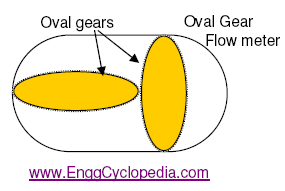Oval gear flow meter is another positive displacement type flow meter. This flow meter uses two or more oblong gears configured to rotate at right angles to one another, forming a T shape. This arrangement allows formation of two section in the flow meter chamber which are completely sealed from each other, much like sections in the oval gear type positive displacement pumps. Thus each rotation of the gear corresponds to a fixed fluid volume passing through the flow meter. Thus flow rate and volume passing through the meter can be measured by monitoring the number of gear rotations.

Rotameter / Variable area meter consists of a vertical tapered glass tube and a float inside the tube.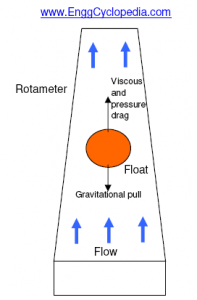The arrangement of tapered tube and float is such that the float is pushed up by the increase in flow due to higher pressure and viscous forces and the float is pulled down by gravity if the flowrate decreases. The rotameter is calibrated to be used for a specific service, typically water or air.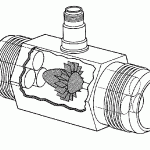Turbine flow meters utilize a turbine wheel for the measurement of flow, as the name suggests. This turbine wheel sent in the path of fluid flow rotates, assisted by the fluid hitting the turbine blades. Speed of rotation is proportional to fluid velocity hitting the turbine blades and the flow meter is calibrated accordingly to accurately measure the flow. They are commonly used in fire water systems and other water distribution systems.

Single jet flow meter and Paddle wheel flow meter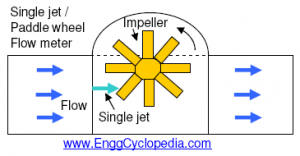use a mechanism similar to turbine flow meters and consist of a simple impeller wheel with radial vanes, impinged upon by a single jet. For paddle wheel flow meters the wheel blades are smaller in width compared to single jet flow meter and are only partially projected in the path of the flow.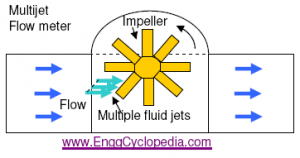Multiple jet flow meter is similar to single jet  flow meter except that the flow is thrown on the impeller blades from multiple points thus hitting multiple points on the circumference of turbine blades. This arrangement minimizes the wear on impeller blades.

Pelton wheel flow meter or Radial turbine flow meter uses a radial turbine to convert the fluid flow into speed of rotation for the turbine wheel which is calibrated to measure fluid velocity and fluid flow.

Nutating disc flow meter uses an eccentrically mounted disc which nutates or wobbles when the fluid flow hits the disc. The tilted disc forms a chamber to allow the fluid flow which remains on one side of the disc. Thus one rotation of the disc corresponds to fixed volume that has passed through. This type is the most commonly used flow meter to measure water supply flow rates.

uses two or more oblong gears configured to rotate at right angles to one another, forming a T shape